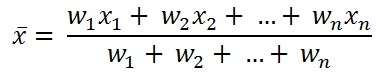## Weighted Mean Calculator

 Enter Value of X= 1,2,3,4,5 Enter the Numbers with Comma separated(,) Enter the Weights to the X values= 5,20,40,80,100 Enter the Numbers with Comma separated(,)
 Result: No.of Inputs Weighted Mean Calculation steps

The Weighted Mean Calculator to calculate the Weighted Mean by giving different weights to some of the individual values.

### Weighted Mean Formulawhere, w is the number of occurrences of x (weight)

x is the repeating value

is the weighted mean

For example, When Value of X = {1,2,3,4,5}, the Weights to the X values = {5,20,40,80,100}

```Weighted mean= (W1X1+W2X2+W3X3+...WnXn)/(W1+W2+W3+...Wn)
Weighted mean=(5+40+120+320+500)/(5+20+40+80+100)
Weighted mean=(985)/(245)
Weighted mean=4.020408163265306
Weighted mean(rounded)=4.0204```
Thinkcalculator.com provides you helpful and handy calculator resources.